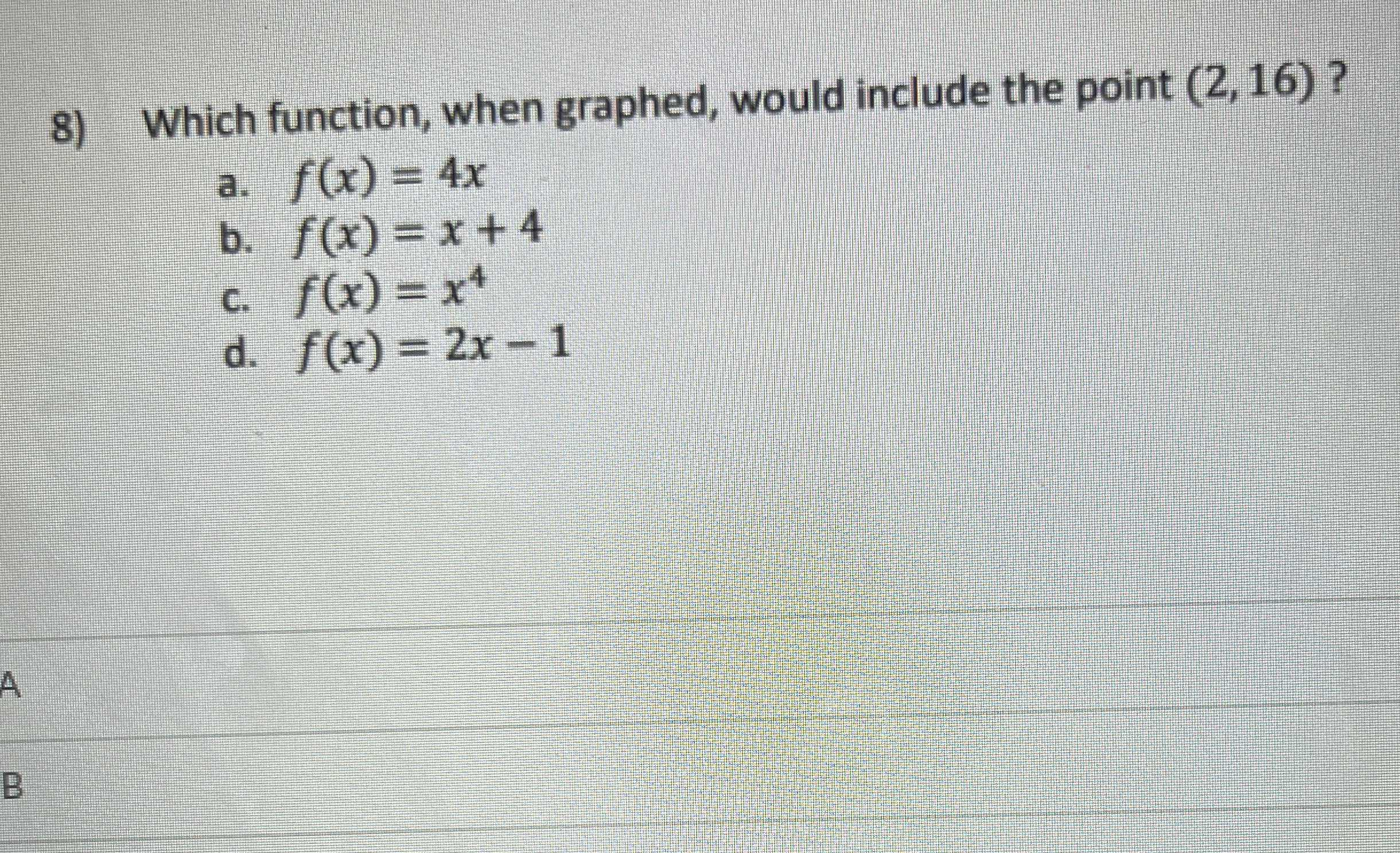### ¿Todavía tienes preguntas de matemáticas?

Pregunte a nuestros tutores expertos
Algebra
Pregunta8) Which function, when graphed, would include the point $$( 2,16 ) ?$$

a. $$f ( x ) = 4 x$$

b. $$f ( x ) = x + 4$$

c. $$f ( x ) = x ^ { 4 }$$

d. $$f ( x ) = 2 x - 1$$